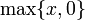# Difference between revisions of "Positive part function"

Jump to: navigation, search

## Definition

The positive part function is a function that takes as input any real number and outputs the same number if it is nonnegative, and 0 if it is negative. The positive part of$x$ is denoted$x^+$. It can also be defined as$\max \{ x, 0 \}$.

It can also be described using a piecewise definition:$x^+ := \left\lbrace \begin{array}{rl}x, & x \ge 0 \\ 0, & x < 0 \\\end{array}\right.$